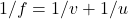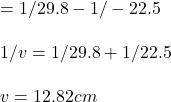## A 2.15 cm tall object is placed in 22.5 cm in front of a concave mirror. The focal length is 29.8 cm. How far is the image from the mi

Question

A 2.15 cm tall object is placed in 22.5 cm in front of a concave mirror. The focal
length is 29.8 cm. How far is the image from the mirror?

in progress 0
2 weeks 2021-08-30T01:17:47+00:00 1 Answers 0 views 0

1. The image is 12.8 cm far from the mirror.

Explanation:

Given:

Height of the object=2.15 cm

To Find:

Distance between the image and mirror.

Solution:

Object distance u=-22.5 cm(object is always placed to the left of the mirror and distances to the left are indicated by negative sign)

Focal length=+29.8 cm(concave mirror has a positive focal length)

The image distance can be calculated using the mirror equation.1/v= 1/f-1/uThus we can conclude that the image is 12.82cm far from the mirror.